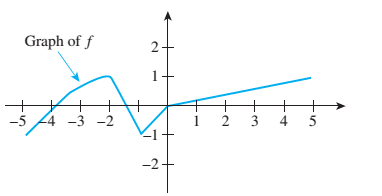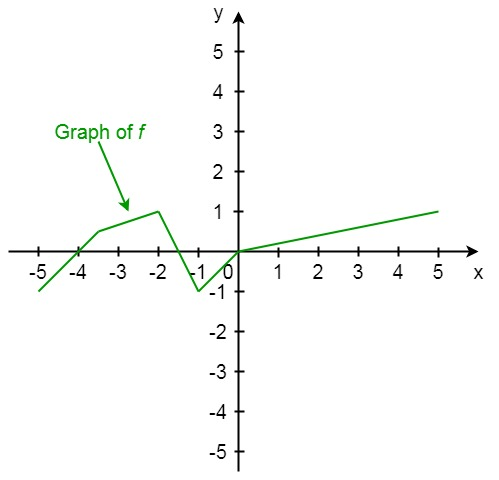Chapter 11.1, Problem 22ES### Discrete Mathematics With Applicat...

5th Edition
EPP + 1 other
ISBN: 9781337694193

#### Solutions

Chapter
Section### Discrete Mathematics With Applicat...

5th Edition
EPP + 1 other
ISBN: 9781337694193
Textbook Problem
1 views

# Let f be the function whose graph follows. Sketch the graph of 3fTo determine

Let f be the function whowse graph is shown below. Draw the graph of 3f.Explanation

Given information:

Let us consider the graph

Concept used:

3f(x)=3(f(x))

Calculation:

Observe that the graph has 5 piecewise straight lines starting from left to right.

Part 1:(5,1),(3.5,0.5)

The line is

y+1=0.5+13.5+5(x+5)y=x+4

Part 2:(3.5,0.5),(2,1)

y0.5=10.52+3.5(x+3.5)y0.5=13(x+3.5)y=13x+53

Part 3:(2,1),(1,1)

y1=111+2(x+2)y1=2(x+2)y=2x3

Part 4:(1,1),(0,0)

y+1=0+10+1(x+1)y=x

Part 5:(0,0),(5,1)

y0=1050(x0)y=15x

Using increasing the above 5 parts by 3 times

### Still sussing out bartleby?

Check out a sample textbook solution.

See a sample solution

#### The Solution to Your Study Problems

Bartleby provides explanations to thousands of textbook problems written by our experts, many with advanced degrees!

Get Started